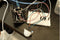# Overview

A passive infrared (PIR) sensor is a type of sensor that detects movement using infrared radiation. Typically, a PIR sensor is used for home automation, motion detection, and many more. In this project, the PIR sensor is used for motion detection, and a light-emitting diode is used as an indicator.

• Arduino IDE

# Application Description

PIR sensors use infrared radiation to detect moving or stationary objects. The PIR sensor is divided into two slots. These slots individually detect infrared radiation from their points of view. When an object moves, the detected infrared radiation is different between the two slots. This creates a differential change between the two slots. On the other hand, there is no change when an object stops moving. This is because the two slots detect the same level of infrared radiation.

In this project, the concept of differential change will be used in detecting motion using a PIR sensor. The PIR sensor is programmed using the Arduino Uno to achieve such feat. Also, a light-emitting diode (LED) is used as an indicator for motion detection. The LED turns on when motion is detected and turns off when motion stops.

# Set-up the HardwareFigure 1 – Schematic DiagramFigure 2 – PIR Motion Sensor Pins

The project can be achieved by following the schematic diagram shown in Figure 1. The pins of the PIR Motion Sensor is shown in Figure 2. The sensor’s three pins (VCC, GND, OUT) are connected to the 5V pin, GND pin, and pin 2 of the Arduino, respectively. The LED (light-emitting diode) is connected to pin 13 of the Arduino. A 330Ω resistor is connected in series to protect the LED.

# Set-up the Software

```int ledPin = 13;                // choose the pin for the LED
int inputPin = 2;               // choose the input pin (for PIR sensor)
int pirState = LOW;             // we start, assuming no motion detected
int val = 0;                    // variable for reading the pin status

void setup() {
pinMode(ledPin, OUTPUT);      // declare LED as output
pinMode(inputPin, INPUT);     // declare sensor as input
}
void loop(){
if (val == HIGH) {            // check if the input is HIGH
digitalWrite(ledPin, HIGH);  // turn LED ON
if (pirState == LOW) {
// we have just turned on
pirState = HIGH;
}
} else {
digitalWrite(ledPin, LOW); // turn LED OFF
if (pirState == HIGH){
// we have just turned off
pirState = LOW;
}
}
}```

The code needed to program the Arduino Uno as a motion detector using the PIR Motion Sensor is shown above.

```int ledPin = 13;                // choose the pin for the LED
int inputPin = 2;               // choose the input pin (for PIR sensor)
int pirState = LOW;             // we start, assuming no motion detected
int val = 0;                    // variable for reading the pin status```

The first part of the code is the initialization and declaration of variables. These variables include the LED pin, sensor pin, initial value of the pin status and the initial state of the PIR sensor.

```void setup() {
pinMode(ledPin, OUTPUT);      // declare LED as output
pinMode(inputPin, INPUT);     // declare sensor as input
}```

The second part is the setup function. This is where the different pins are assigned as either outputs or inputs. In this project, the LED pin is used as an output while the sensor pin is assigned as an input.

```void loop(){
if (val == HIGH) {            // check if the input is HIGH
digitalWrite(ledPin, HIGH);  // turn LED ON
if (pirState == LOW) {
// we have just turned on
pirState = HIGH;
}
} else {
digitalWrite(ledPin, LOW); // turn LED OFF
if (pirState == HIGH){
// we have just turned off
pirState = LOW;
}
}
}```

The third and final part of the Arduino code is the loop function. This is where the Arduino does its processes and repeats it continuously. This project uses the if-else statement to program the Arduino. After reading the input value, the code uses a nested-if statement and an if-else statement. When the input value is in a high state, the LED pin gives off a high signal, which allows the LED to turn on. On the other hand, the LED turns off when the input value is in a low state. Also, the nested-if statement is used to check the state of the sensor, and to correct it depending on the input value.

# Conclusion

The PIR sensor is used as a motion detector, as shown above. However, this is not the only project that the PIR sensor is used. You may use your creativity and imagination. This is only the beginning!

# Reference

The post Motion Detector using PIR Module appeared first on CreateLabz.